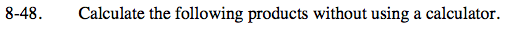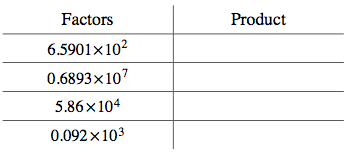Home > ACC7 > Chapter cc38 > Lesson cc38.2.1 > Problem8-48

8-48.
1. Calculate the following products without using a calculator. Homework Help ✎2.  Factors Product 6.5901 × 102 0.6893 × 107 5.86 × 104 0.092 × 103For help on multiplying numbers by powers of 10 and moving the decimal point, see problem 8-40.

Two of the table's solutions are provided below.
Complete the rest of the table.

0.6893 × 107 = 6,893,000

0.092 × 103 = 92